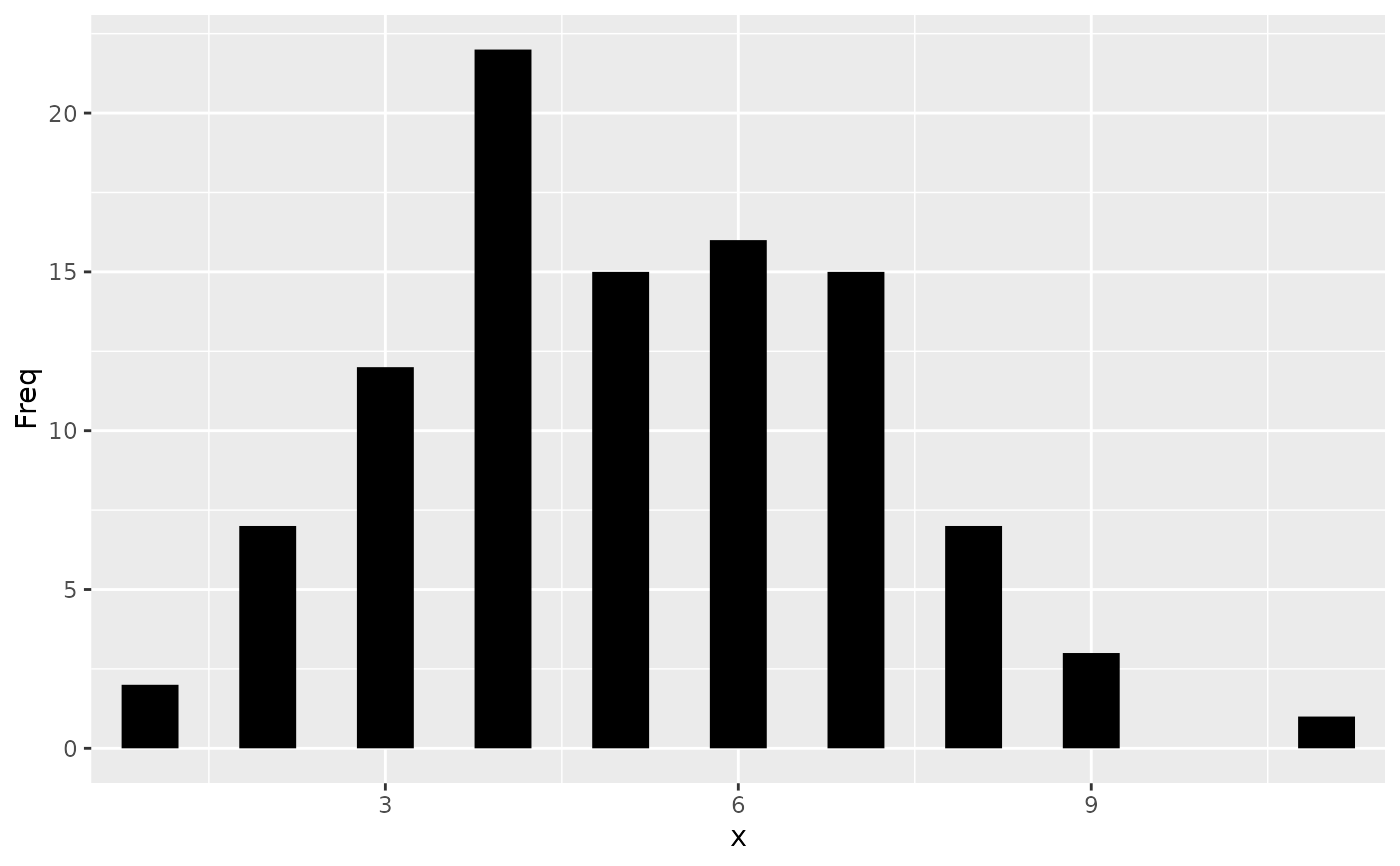The following aesthetics can be used to specify the position of elements: x, y, xmin, xmax, ymin, ymax, xend, yend.

## Details

x and y define the locations of points or of positions along a line or path.

x, y and xend, yend define the starting and ending points of segment and curve geometries.

xmin, xmax, ymin and ymax can be used to specify the position of annotations and to represent rectangular areas.

In addition, there are position aesthetics that are contextual to the geometry that they're used in. These are xintercept, yintercept, xmin_final, ymin_final, xmax_final, ymax_final, xlower, lower, xmiddle, middle, xupper, upper, x0 and y0. Many of these are used and automatically computed in geom_boxplot().

• Geoms that commonly use these aesthetics: geom_crossbar(), geom_curve(), geom_errorbar(), geom_line(), geom_linerange(), geom_path(), geom_point(), geom_pointrange(), geom_rect(), geom_segment()

• Scales that can be used to modify positions: scale_continuous(), scale_discrete(), scale_binned(), scale_date().

• See also annotate() for placing annotations.

Other aesthetics documentation: aes_colour_fill_alpha, aes_group_order, aes_linetype_size_shape, aes()

## Examples


# Generate data: means and standard errors of means for prices
# for each type of cut
dmod <- lm(price ~ cut, data = diamonds)
cut <- unique(diamonds$cut) cuts_df <- data.frame( cut, predict(dmod, data.frame(cut), se = TRUE)[c("fit", "se.fit")] ) ggplot(cuts_df) + aes( x = cut, y = fit, ymin = fit - se.fit, ymax = fit + se.fit, colour = cut ) + geom_pointrange()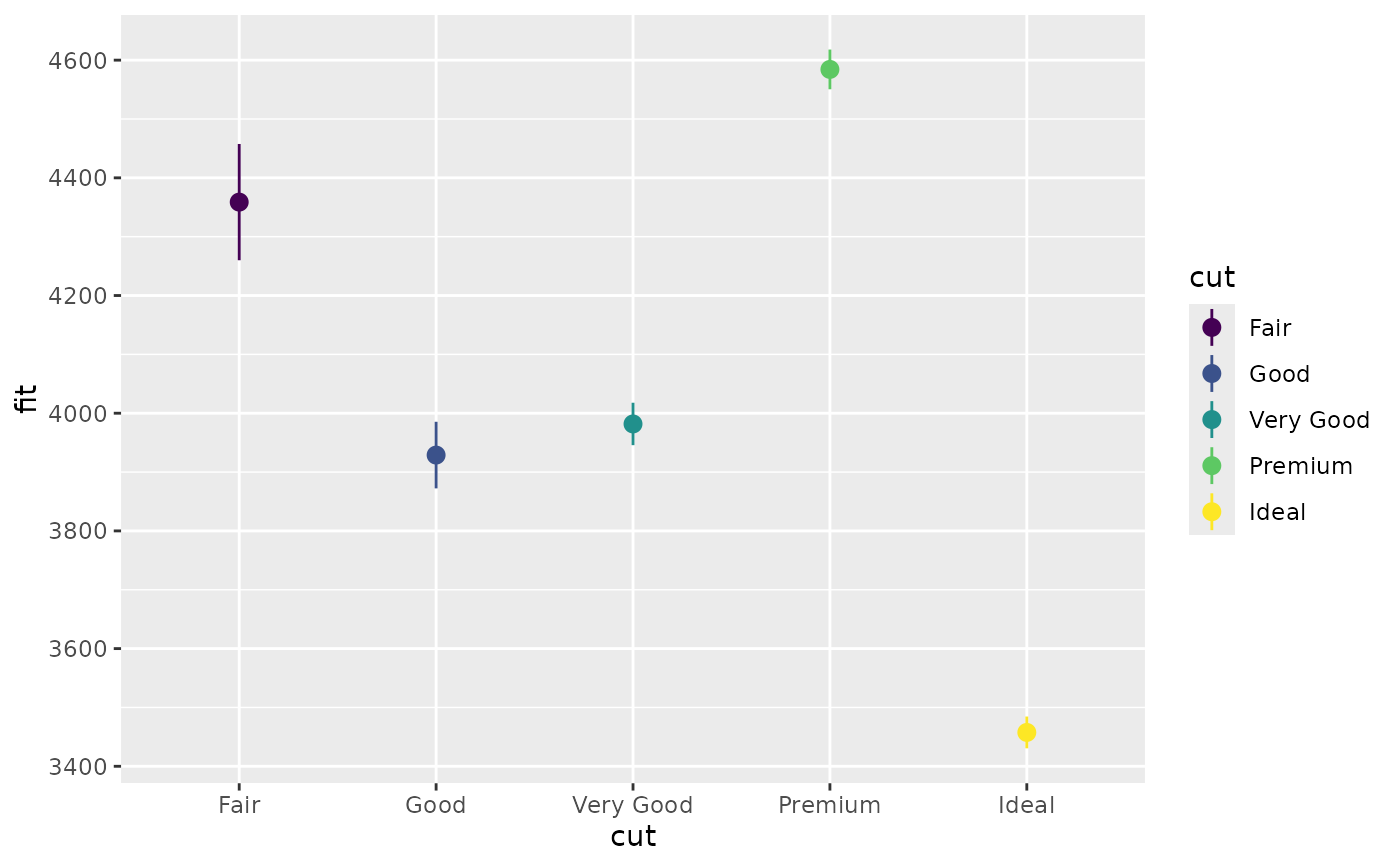# Using annotate p <- ggplot(mtcars, aes(x = wt, y = mpg)) + geom_point() p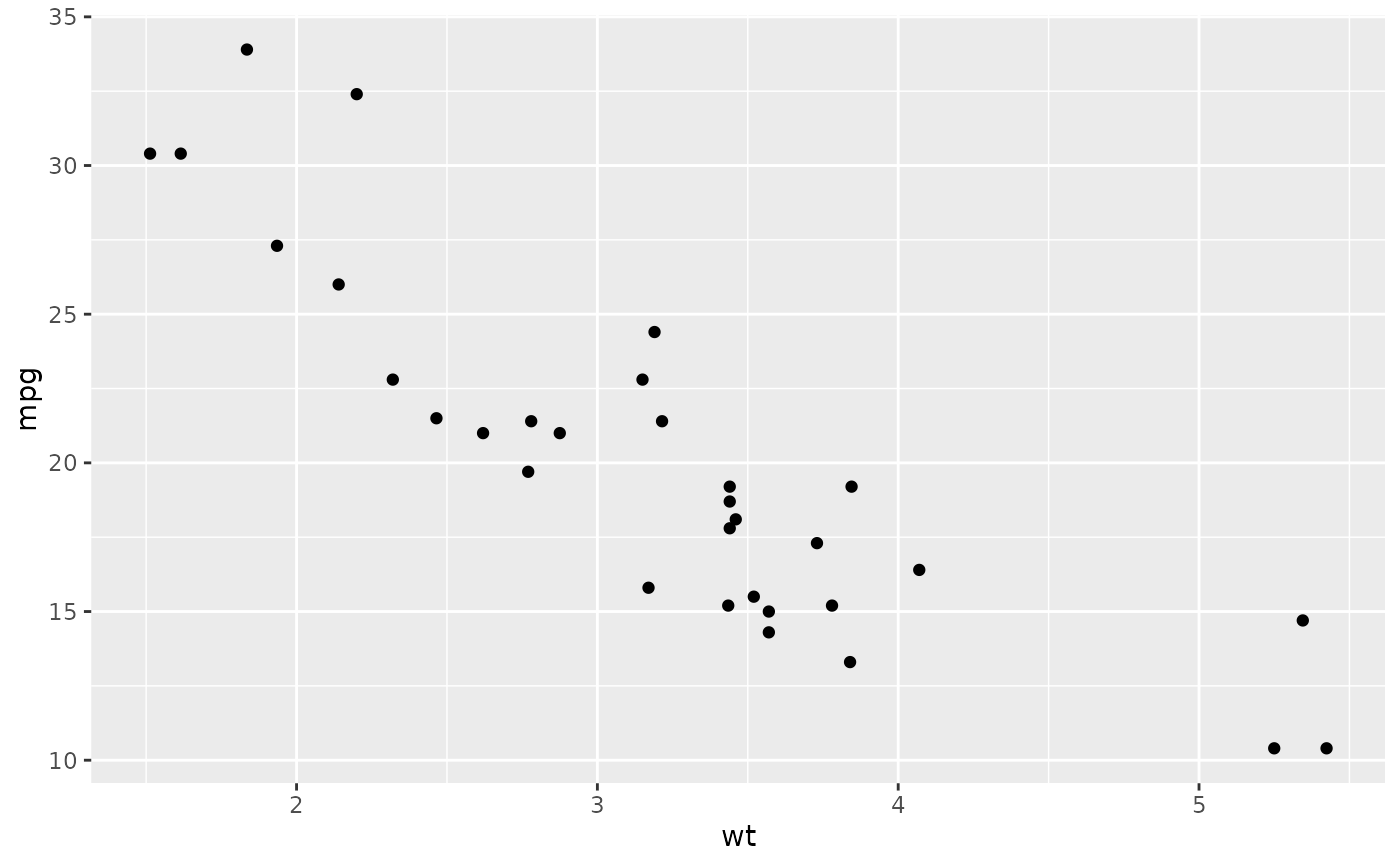p + annotate( "rect", xmin = 2, xmax = 3.5, ymin = 2, ymax = 25, fill = "dark grey", alpha = .5 )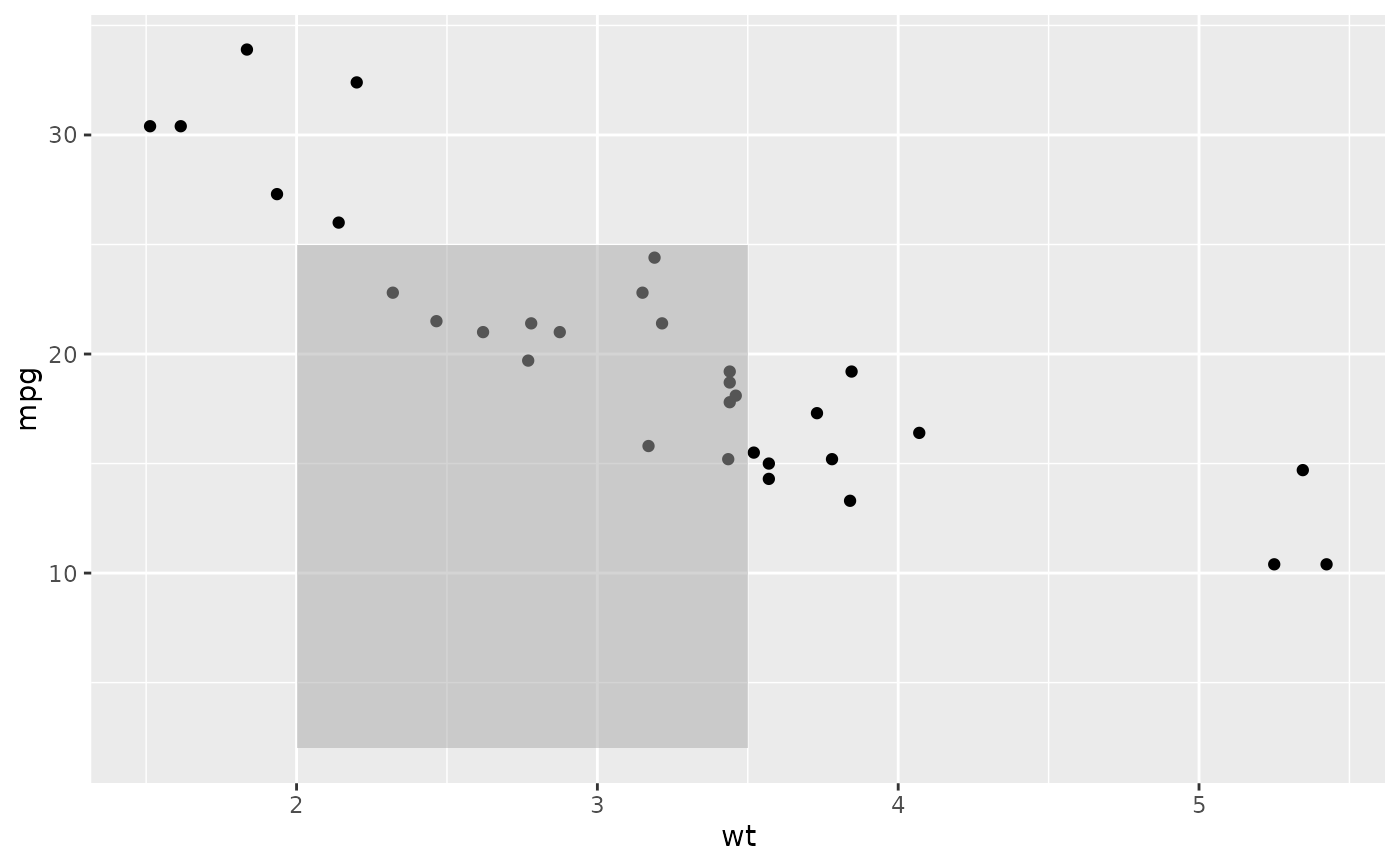# Geom_segment examples p + geom_segment( aes(x = 2, y = 15, xend = 2, yend = 25), arrow = arrow(length = unit(0.5, "cm")) ) #> Warning: All aesthetics have length 1, but the data has 32 rows. #> ℹ Did you mean to use annotate()?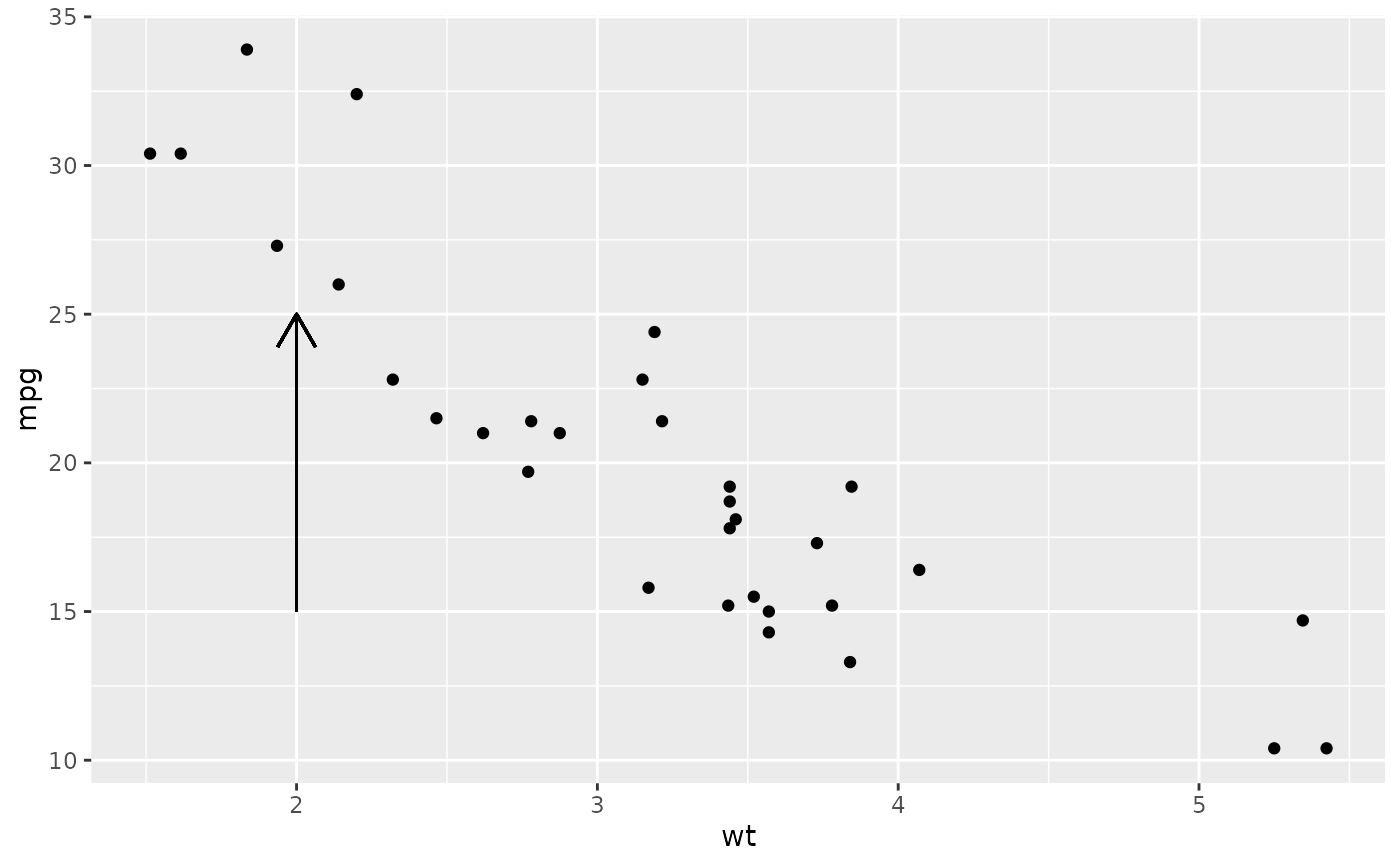p + geom_segment( aes(x = 2, y = 15, xend = 3, yend = 15), arrow = arrow(length = unit(0.5, "cm")) ) #> Warning: All aesthetics have length 1, but the data has 32 rows. #> ℹ Did you mean to use annotate()?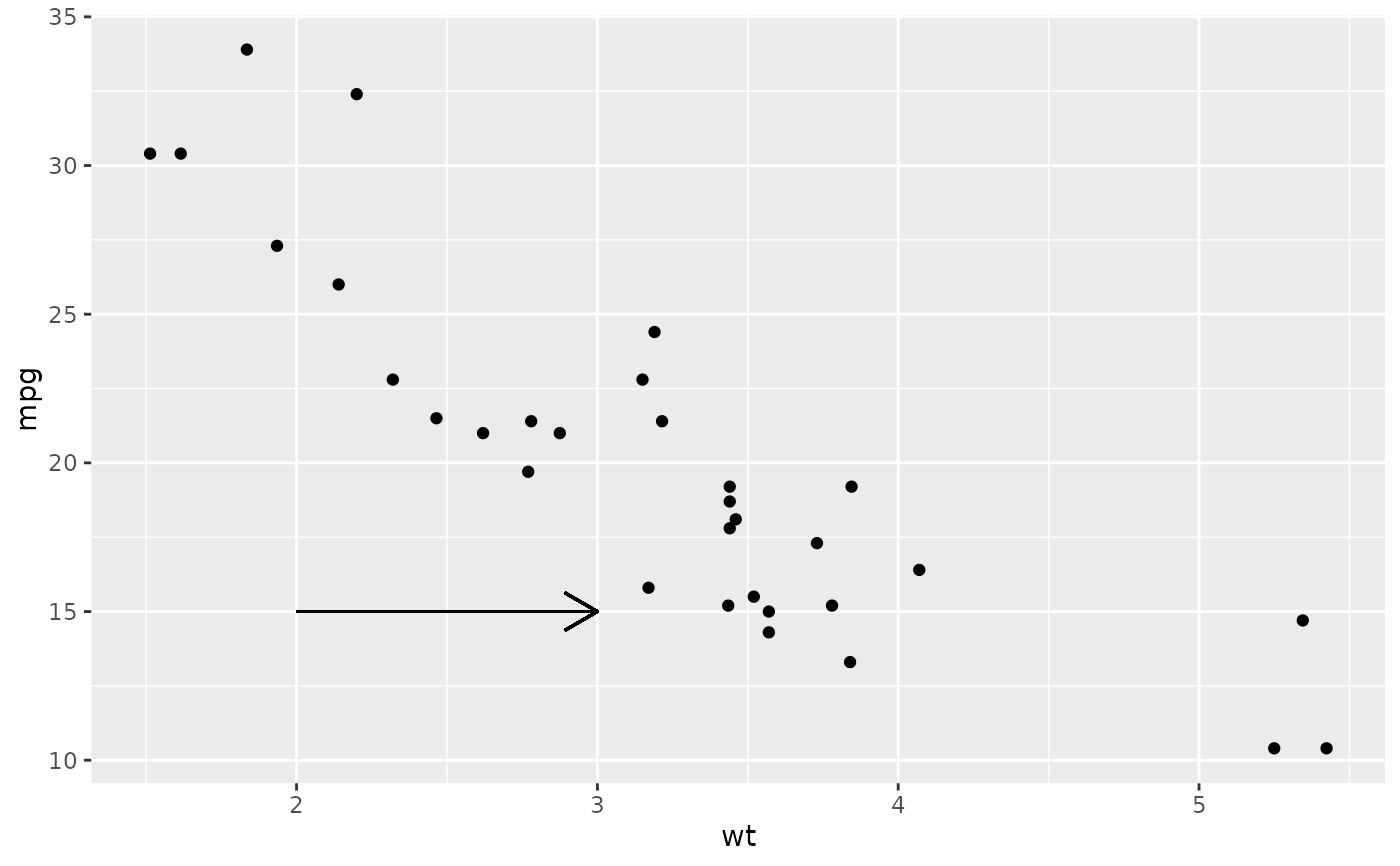p + geom_segment( aes(x = 5, y = 30, xend = 3.5, yend = 25), arrow = arrow(length = unit(0.5, "cm")) ) #> Warning: All aesthetics have length 1, but the data has 32 rows. #> ℹ Did you mean to use annotate()?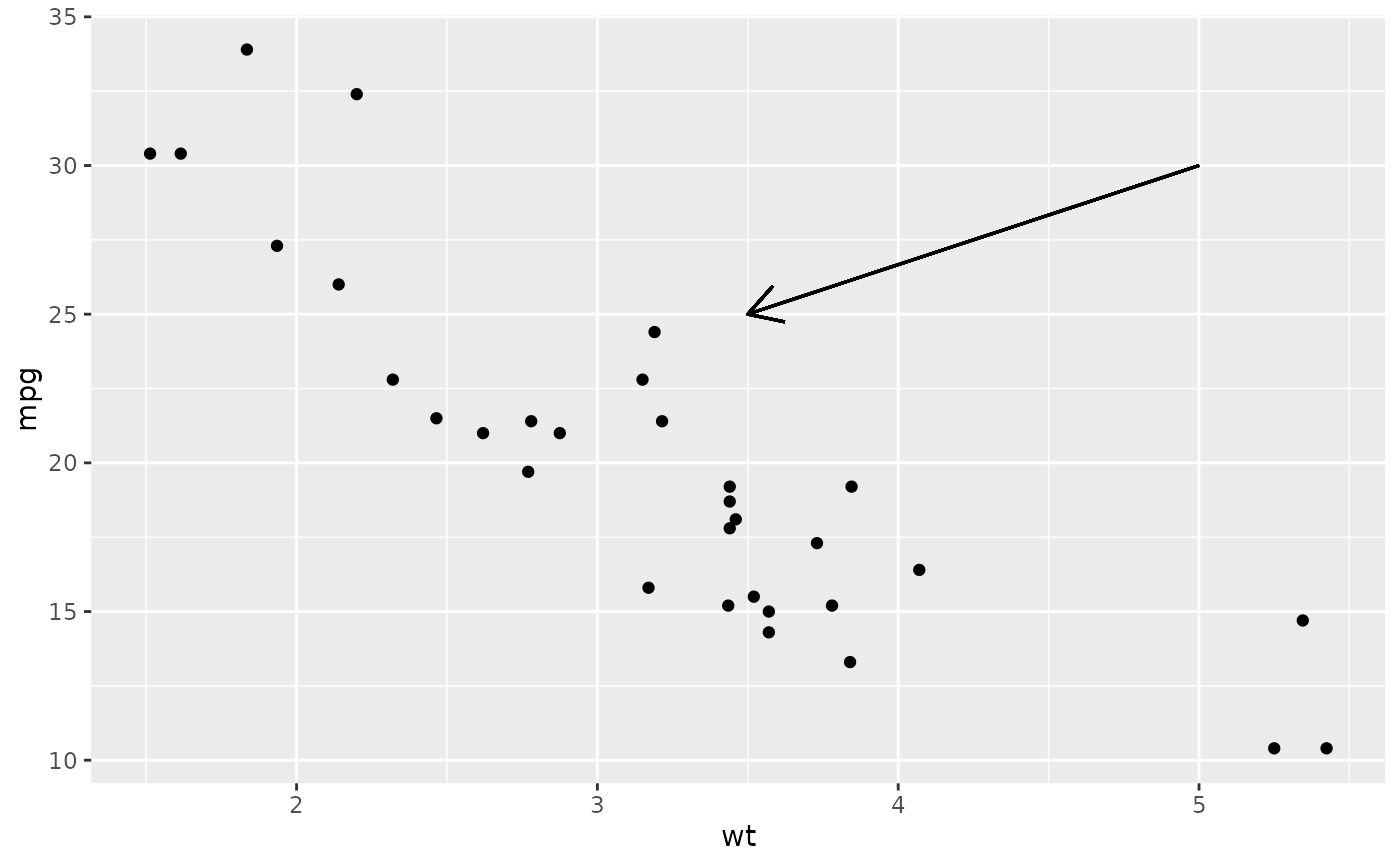# You can also use geom_segment() to recreate plot(type = "h") # from base R: set.seed(1) counts <- as.data.frame(table(x = rpois(100, 5))) counts$x <- as.numeric(as.character(counts\$x))
with(counts, plot(x, Freq, type = "h", lwd = 10))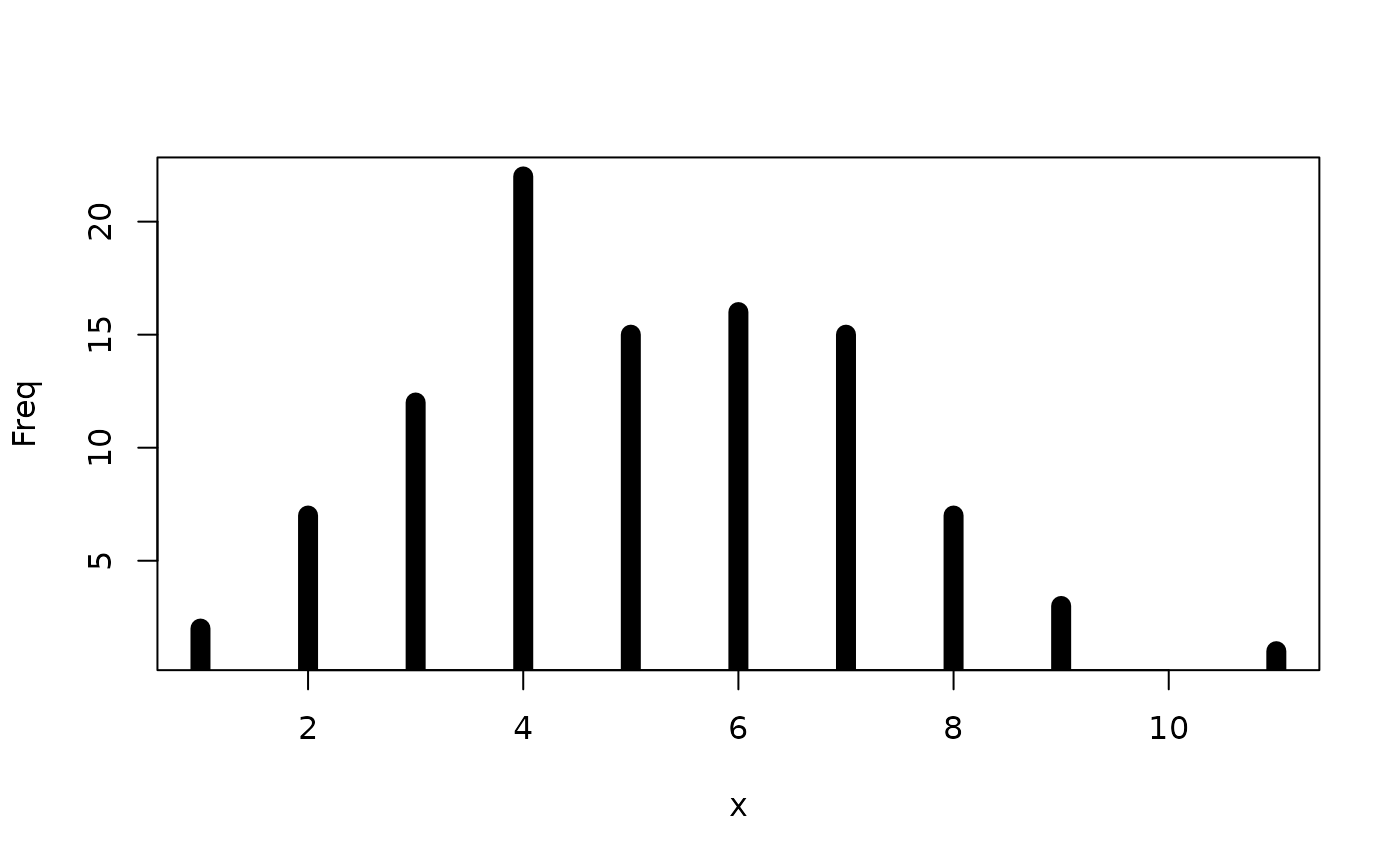ggplot(counts, aes(x = x, y = Freq)) +
geom_segment(aes(yend = 0, xend = x), size = 10)